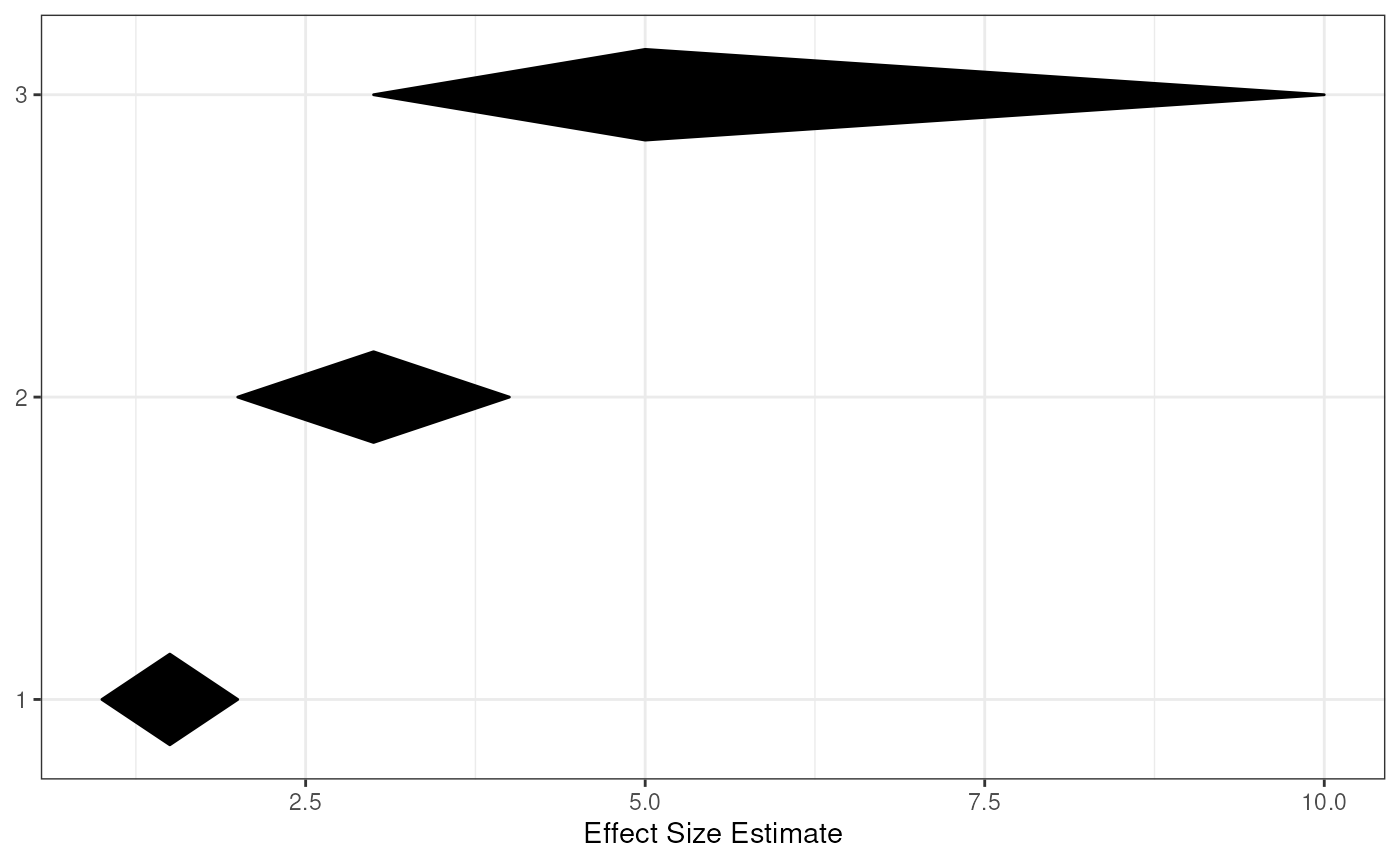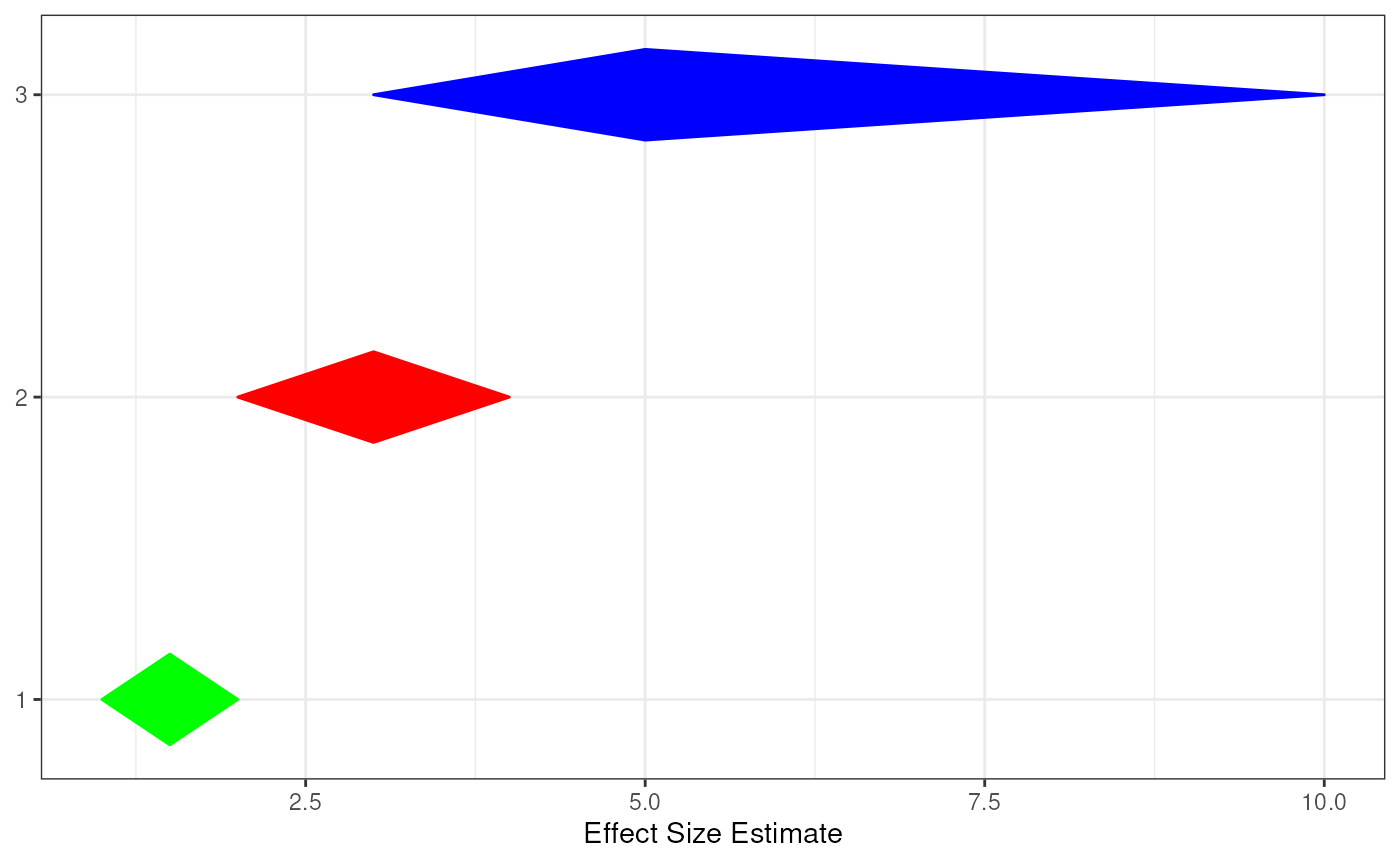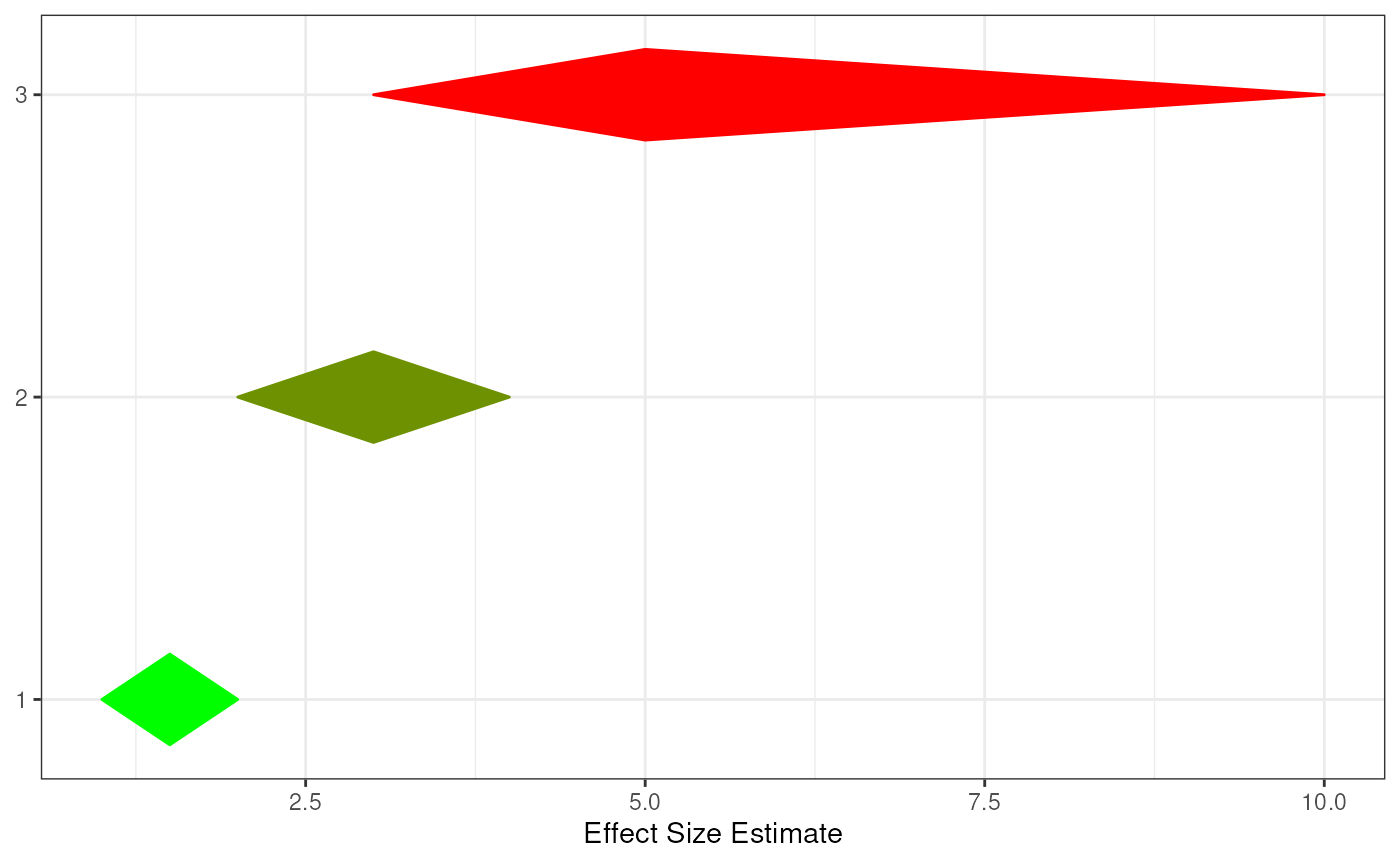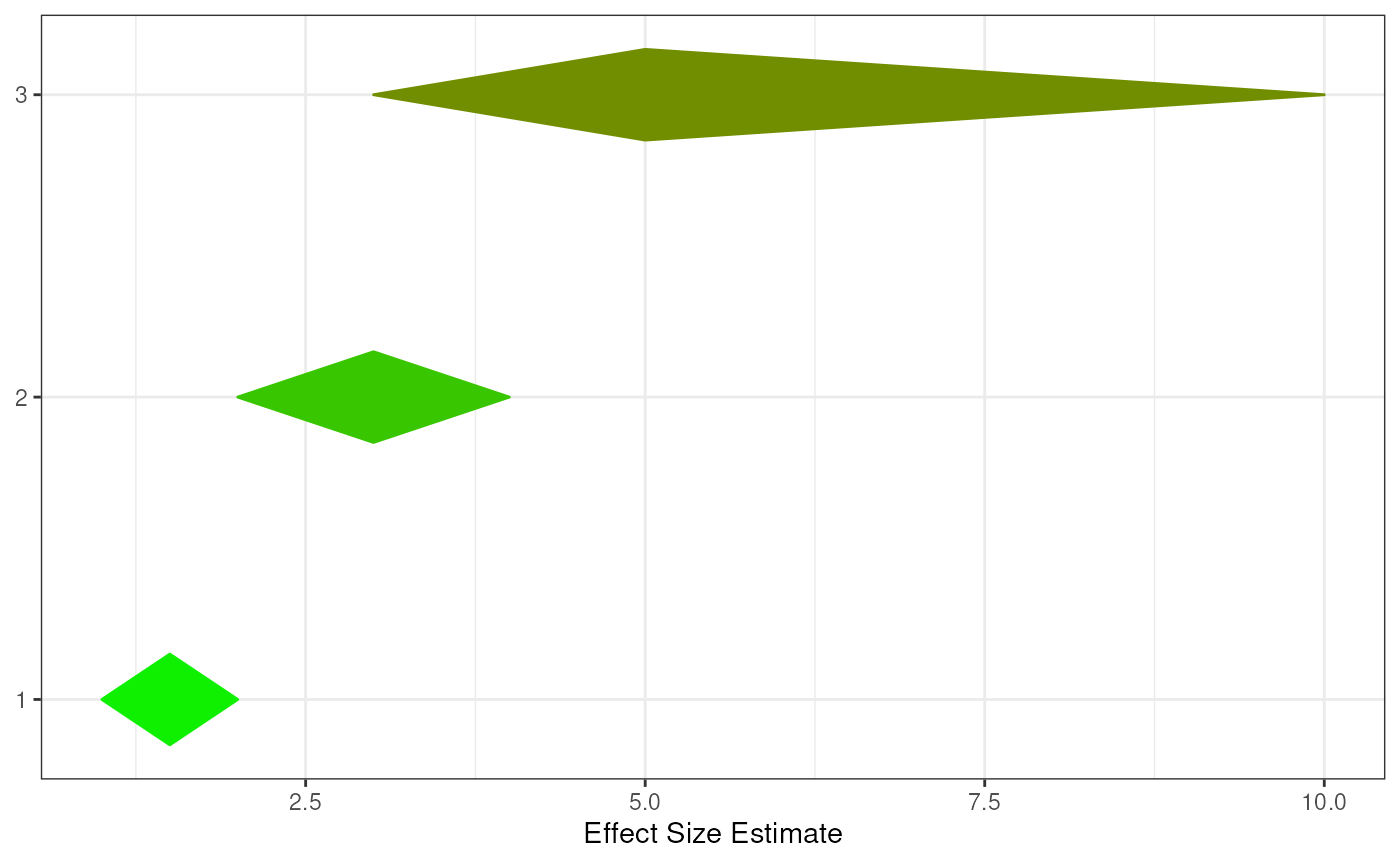This function constructs a diamond plot using ggDiamondLayer(). It's normally not necessary to call this function directly: instead, use meansDiamondPlot() meanSDtoDiamondPlot(), and factorLoadingDiamondCIplot().

diamondPlot(
data,
ciCols = 1:3,
colorCol = NULL,
otherAxisCol = NULL,
yValues = NULL,
yLabels = NULL,
ylab = NULL,
autoSize = NULL,
fixedSize = 0.15,
xlab = "Effect Size Estimate",
theme = ggplot2::theme_bw(),
color = "black",
returnLayerOnly = FALSE,
outputFile = NULL,
outputWidth = 10,
outputHeight = 10,
ggsaveParams = ufs::opts\$get("ggsaveParams"),
...
)

## Arguments

data

A dataframe (or matrix) containing lower bounds, centers (e.g. means), and upper bounds of intervals (e.g. confidence intervals).

ciCols

The columns in the dataframe with the lower bounds, centers (e.g. means), and upper bounds (in that order).

colorCol

The column in the dataframe containing the colors for each diamond, or a vector with colors (with as many elements as the dataframe has rows).

otherAxisCol

The column in the dataframe containing the values that determine where on the Y axis the diamond should be placed. If this is not available in the dataframe, specify it manually using yValues.

yValues

The values that determine where on the Y axis the diamond should be placed (can also be a column in the dataframe; in that case, use otherAxisCol.

yLabels

The labels to use for for each diamond (placed on the Y axis).

autoSize

Whether to make the height of each diamond conditional upon its length (the width of the confidence interval).

fixedSize

If not using relative heights, fixedSize determines the height to use.

xlab, ylab

The labels of the X and Y axes.

theme

The theme to use.

color

Color to use if colors are specified for each diamond.

returnLayerOnly

Set this to TRUE to only return the ggplot() layer of the diamondplot, which can be useful to include it in other plots.

outputFile

A file to which to save the plot.

outputWidth, outputHeight

Width and height of saved plot (specified in centimeters by default, see ggsaveParams).

ggsaveParams

Parameters to pass to ggsave when saving the plot.

...

Additional arguments will be passed to ggDiamondLayer().

## Value

A ggplot2::ggplot() plot with a ggDiamondLayer() is returned.

meansDiamondPlot(), meanSDtoDiamondPlot(), ggDiamondLayer(), factorLoadingDiamondCIplot()

## Author

Gjalt-Jorn Peters

Maintainer: Gjalt-Jorn Peters gjalt-jorn@userfriendlyscience.com

## Examples


tmpDf <- data.frame(lo = c(1, 2, 3),
mean = c(1.5, 3, 5),
hi = c(2, 4, 10),
color = c('green', 'red', 'blue'));

### A simple diamond plot
diamondPlot(tmpDf);### A diamond plot using the specified colours
diamondPlot(tmpDf, colorCol = 4);### A diamond plot using automatically generated colours